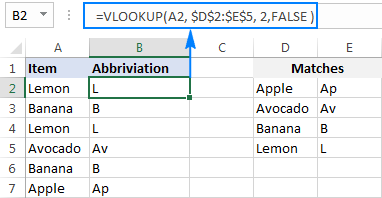# Excel: If cell contains formula examples

The tutorial provides a number of "Excel if contains" formula examples that show how to return something in another column if a target cell contains a required value, how to search with partial match and test multiple criteria with OR as well as AND logic.

One of the most common tasks in Excel is checking whether a cell contains a value of interest. What kind of value can that be? Just any text or number, specific text, or any value at all (not empty cell).

There exist several variations of "If cell contains" formula in Excel, depending on exactly what values you want to find. Generally, you will use the IF function to do a logical test, and return one value when the condition is met (cell contains) and/or another value when the condition is not met (cell does not contain). The below examples cover the most frequent scenarios.

## If cell contains any value, then

For starters, let's see how to find cells that contain anything at all: any text, number, or date. For this, we are going to use a simple IF formula that checks for non-blank cells.

IF(cell<>"", value_to_return, "")

For example, to return "Not blank" in column B if column A's cell in the same row contains any value, you enter the following formula in B2, and then double click the small green square in the lower-right corner to copy the formula down the column:

`=IF(A2<>"", "Not blank", "")`

The result will look similar to this:## If cell contains text, then

If you want to find only cells with text values ignoring numbers and dates, then use IF in combination with the ISTEXT function. Here's the generic formula to return some value in another cell if a target cell contains any text:

IF(ISTEXT(cell), value_to_return, "")

Supposing, you want to insert the word "yes" in column B if a cell in column A contains text. To have it done, put the following formula in B2:

`=IF(ISTEXT(A2), "Yes", "")`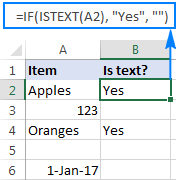## If cell contains number, then

In a similar fashion, you can identify cells with numeric values (numbers and dates). For this, use the IF function together with ISNUMBER:

IF(ISNUMBER(cell), value_to_return, "")

The following formula returns "yes" in column B if a corresponding cell in column A contains any number:

`=IF(ISNUMBER(A2), "Yes", "")`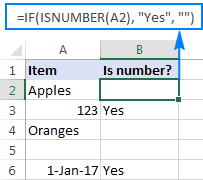## If cell contains specific text

Finding cells containing certain text (or numbers or dates) is easy. You write a regular IF formula that checks whether a target cell contains the desired text, and type the text to return in the value_if_true argument.

IF(cell="text", value_to_return, "")

For example, to find out if cell A2 contains "apples", use this formula:

`=IF(A2="apples", "Yes", "")`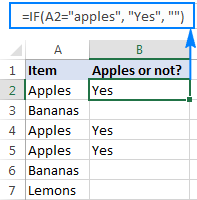### If cell does not contain specific text

If you are looking for the opposite result, i.e. return some value to another column if a target cell does not contain the specified text ("apples"), then do one of the following.

Supply an empty string ("") in the value_if_true argument, and text to return in the value_if_false argument:

`=IF(A2="apples", "", "Not apples")`

Or, put the "not equal to" operator in logical_test and text to return in value_if_true:

`=IF(A2<>"apples", "Not apples", "")`

Either way, the formula will produce this result: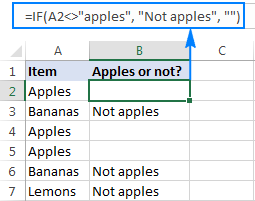### If cell contains text: case-sensitive formula

To force your formula to distinguish between uppercase and lowercase characters, use the EXACT function that checks whether two text strings are exactly equal, including the letter case:

`=IF(EXACT(A2,"APPLES"), "Yes", "")`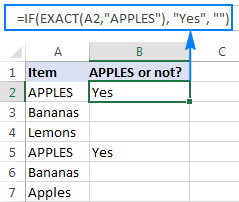You can also input the model text string in some cell (say in C1), fix the cell reference with the \$ sign (\$C\$1), and compare the target cell with that cell:

`=IF(EXACT(A2,\$C\$1), "Yes", "")`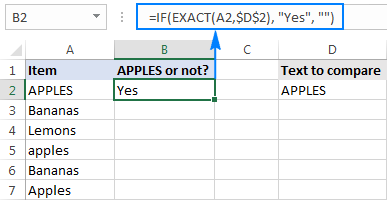## If cell contains specific text string (partial match)

We have finished with trivial tasks and move on to more challenging and interesting ones :) In this example, it takes three different functions to find out whether a given character or substring is part of the cell contents:

IF(ISNUMBER(SEARCH("text", cell)), value_to_return,"")

Working from the inside out, here is what the formula does:

• The SEARCH function searches for a text string, and if the string is found, returns the position of the first character, the #VALUE! error otherwise.
• The ISNUMBER function checks whether SEARCH succeeded or failed. If SEARCH has returned any number, ISNUMBER returns TRUE. If SEARCH results in an error, ISNUMBER returns FALSE.
• Finally, the IF function returns the specified value for cells that have TRUE in the logical test, an empty string ("") otherwise.

And now, let's see how this generic formula works in real-life worksheets.

### If cell contains certain text, put a value in another cell

Supposing you have a list of orders in column A and you want to find orders with a specific identifier, say "A-". The task can be accomplished with this formula:

`=IF(ISNUMBER(SEARCH("A-",A2)),"Valid","")`

Instead of hardcoding the string in the formula, you can input it in a separate cell (E1), the reference that cell in your formula:

`=IF(ISNUMBER(SEARCH(\$E\$1,A2)),"Valid","")`

For the formula to work correctly, be sure to lock the address of the cell containing the string with the \$ sign (absolute cell reference).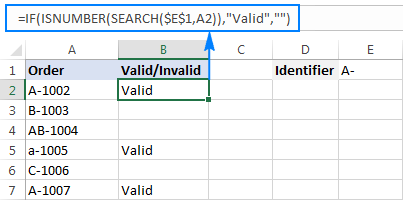### If cell contains specific text, copy it to another column

If you wish to copy the contents of the valid cells somewhere else, simply supply the address of the evaluated cell (A2) in the value_if_true argument:

`=IF(ISNUMBER(SEARCH(\$E\$1,A2)),A2,"")`

The screenshot below shows the results: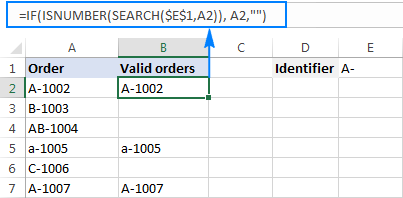### If cell contains specific text: case-sensitive formula

In both of the above examples, the formulas are case-insensitive. In situations when you work with case-sensitive data, use the FIND function instead of SEARCH to distinguish the character case.

For example, the following formula will identify only orders with the uppercase "A-" ignoring lowercase "a-".

`=IF(ISNUMBER(FIND("A-",A2)),"Valid","")`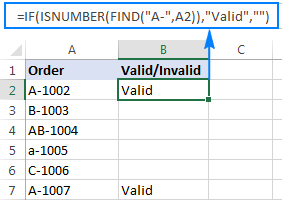## If cell contains one of many text strings (OR logic)

To identify cells containing at least one of many things you are searching for, use one of the following formulas.

### IF OR ISNUMBER SEARCH formula

The most obvious approach would be to check for each substring individually and have the OR function return TRUE in the logical test of the IF formula if at least one substring is found:

IF(OR(ISNUMBER(SEARCH("string1", cell)), ISNUMBER(SEARCH("string2", cell))), value_to_return, "")

Supposing you have a list of SKUs in column A and you want to find those that include either "dress" or "skirt". You can have it done by using this formula:

`=IF(OR(ISNUMBER(SEARCH("dress",A2)),ISNUMBER(SEARCH("skirt",A2))),"Valid ","")`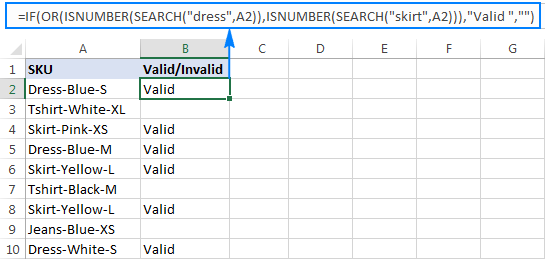The formula works pretty well for a couple of items, but it's certainly not the way to go if you want to check for many things. In this case, a better approach would be using the SUMPRODUCT function as shown in the next example.

### SUMPRODUCT ISNUMBER SEARCH formula

If you are dealing with multiple text strings, searching for each string individually would make your formula too long and difficult to read. A more elegant solution would be embedding the ISNUMBER SEARCH combination into the SUMPRODUCT function, and see if the result is greater than zero:

SUMPRODUCT(--ISNUMBER(SEARCH(strings, cell)))>0

For example, to find out if A2 contains any of the words input in cells D2:D4, use this formula:

`=SUMPRODUCT(--ISNUMBER(SEARCH(\$D\$2:\$D\$4,A2)))>0`

Alternatively, you can create a named range containing the strings to search for, or supply the words directly in the formula:

`=SUMPRODUCT(--ISNUMBER(SEARCH({"dress","skirt","jeans"},A2)))>0`

Either way, the result will be similar to this: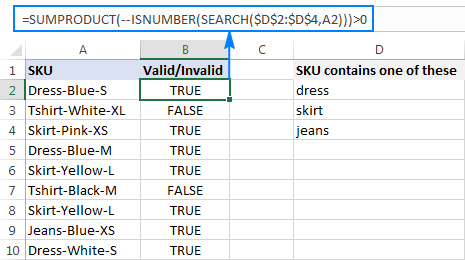To make the output more user-friendly, you can nest the above formula into the IF function and return your own text instead of the TRUE/FALSE values:

`=IF(SUMPRODUCT(--ISNUMBER(SEARCH(\$D\$2:\$D\$4,A2)))>0, "Valid", "")`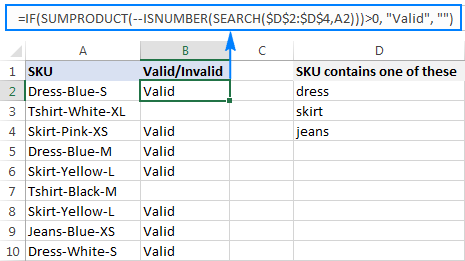#### How this formula works

At the core, you use ISNUMBER together with SEARCH as explained in the previous example. In this case, the search results are represented in the form of an array like {TRUE;FALSE;FALSE}. If a cell contains at least one of the specified substrings, there will be TRUE in the array. The double unary operator (--) coerces the TRUE / FALSE values to 1 and 0, respectively, and delivers an array like {1;0;0}. Finally, the SUMPRODUCT function adds up the numbers, and we pick out cells where the result is greater than zero.

## If cell contains several strings (AND logic)

In situations when you want to find cells containing all of the specified text strings, use the already familiar ISNUMBER SEARCH combination together with IF AND:

IF(AND(ISNUMBER(SEARCH("string1",cell)), ISNUMBER(SEARCH("string2",cell))), value_to_return,"")

For example, you can find SKUs containing both "dress" and "blue" with this formula:

`=IF(AND(ISNUMBER(SEARCH("dress",A2)),ISNUMBER(SEARCH("blue",A2))),"Valid ","")`

Or, you can type the strings in separate cells and reference those cells in your formula:

`=IF(AND(ISNUMBER(SEARCH(\$D\$2,A2)),ISNUMBER(SEARCH(\$E\$2,A2))),"Valid ","")`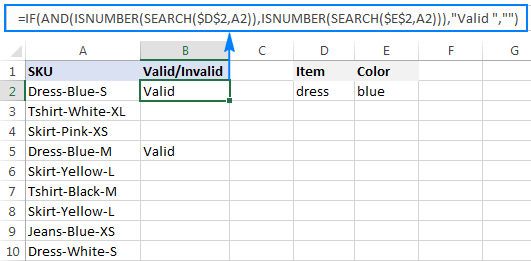As an alternative solution, you can count the occurrences of each string and check if each count is greater than zero:

`=IF(AND(COUNTIF(A2,"*dress*")>0,COUNTIF(A2,"*blue*")>0),"Valid","")`

The result will be exactly like shown in the screenshot above.

## How to return different results based on cell value

In case you want to compare each cell in the target column against another list of items and return a different value for each match, use one of the following approaches.

### Nested IFs

The logic of the nested IF formula is as simple as this: you use a separate IF function to test each condition, and return different values depending on the results of those tests.

IF(cell="lookup_text1", "return_text1", IF(cell="lookup_text2", "return_text2", IF(cell="lookup_text3", "return_text3", "")))

Supposing you have a list of items in column A and you want to have their abbreviations in column B. To have it done, use the following formula:

`=IF(A2="apple", "Ap", IF(A2="avocado", "Av", IF(A2="banana", "B", IF(A2="lemon", "L", ""))))`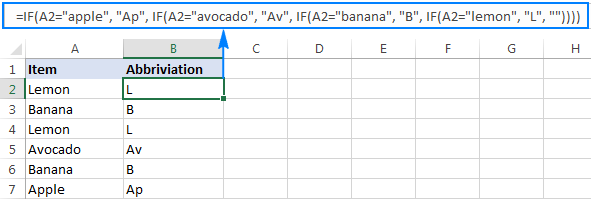For full details about nested IF's syntax and logic, please see Excel nested IF - multiple conditions in a single formula.

### Lookup formula

If you are looking for a more compact and better understandable formula, use the LOOKUP function with lookup and return values supplied as vertical array constants:

LOOKUP(cell, {"lookup_text1";"lookup_text2";"lookup_text3";…}, {"return_text1";"return_text2";"return_text3";…})

For accurate results, be sure to list the lookup values in alphabetical order, from A to Z.

`=LOOKUP(A2,{"apple";"avocado";"banana";"lemon"},{"Ap";"Av";"B";"L"})`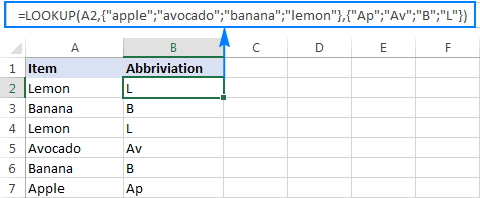Compared to nested IFs, the Lookup formula has one more advantage - it understands the wildcard characters and therefore can identify partial matches.

For example, if column A contains a few sorts of bananas, you can look up "*banana*" and have the same abbreviation ("B") returned for all such cells:

`=LOOKUP(A2,{"apple";"avocado";"*banana*";"lemon"},{"Ap";"Av";"B";"L"})`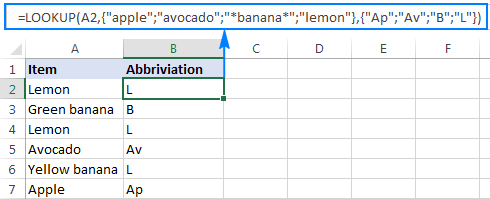### Vlookup formula

When working with a variable data set, it may be more convenient to input a list of matches in separate cells and retrieve them by using a Vlookup formula, e.g.:

`=VLOOKUP(A2, \$D\$2:\$E\$5, 2,FALSE )`